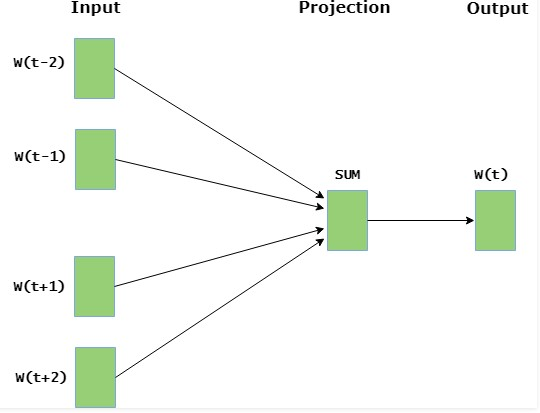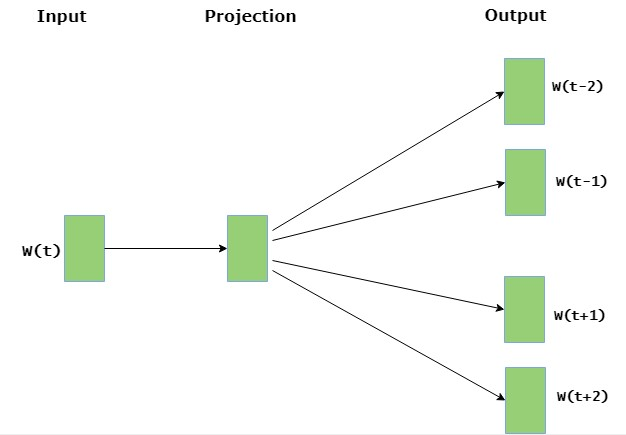# work2vec Word Vector on Python

Work2vec word vector application summary

Under the premise which the model has been acquired:

1. Get the word vector of each word.

model [‘PC’]  #raw Numpy vector of a word.

1. Support the addition and subtraction of words. In case, there may be only some examples which are more consistent.

model.most_similar (positive = [‘man’, ‘queen’], negative=[‘woman’])

1. Calculate the cosine distance between two words

Model.similarity (“nice”, “pretty”)

1. Calculate the ten words with the cosine distance closest to “word”, or topn words.

model.most_similar (“word”)

Model.similar_by_word (‘corruption’, topn =100) The closest 100 words

1. Calculate the cosine likeness between two sets.

When a word is not in the training set, an error is going to be reported.

list_sim1 =  model.n_similarity (list1, list2)

1. Choose the words of different classes in the collection

model.doesnt_match(“breakfast bread dinner lunch”. split ())

Python | Word Embedding by using Word2Vec

Word Embedding is a language modeling technique that is used for mapping the words to vectors of the real numbers. It represents words or phrases in vector space with some dimensions. Word embeddings are able to be generated by using some different ways like neural networks, probabilistic models, co-occurrence matrix, etc.

Word2Vec consists of models for producing word embedding. Those models are shallow two layer neural networks having one input layer; one hidden layer and one output layer. For your information, Word2Vec uses two architectures:

1. CBOW (Continuous Bag of Words)
2. Skip Gram

CBOW (Continuous Bag of Words)CBOW model predicts the current word given the context words within a specific window. For your information, the input layer consists of the context words, while the output layer consists of the current word. The hidden layer contains the number of dimensions in which we wish to represent the current word present at the output layer.

Skip GramSkip gram predicts the surrounding context words within a specific window given the word. The input layer consists of the current word, while the output layer consists of the context words. The hidden layer contains the number of dimensions in which we wish to represent the current word present at the input layer.

You have to note that The basic idea of word embedding is words which occur in similar contexts tend to be closer to each other in vector space. For producing word vectors in Python, modules required are gensim and nltk.

Now, try to run the commands below in terminal to install gensim and nltk:

pip install gensim

pip install nltk

Output

Output specifies the cosine similarities between the word vectors ‘machines, alice’, and ‘wonderland’ for different models. An interesting task which is able to change the parameter values of the size and window to monitor the variations in the cosine similarities.

Develop Word2Vec Embedding

Word2vec is an algorithm for studying a word embedding from a text corpus. Apparently, there are two main training algorithms which are able to be used for learning the embedding from text. They are CBOW (continuous bag of words) and Skip grams. According to the research, the Word2vec approach was built by Tomas Mikolov.

Word2Vec models need a lot of text. For example, the entire Wikipedia corpus. However, we are going to demonstrate the principles by using a small in-memory instance of the text.

Remember that Gensim gives the Word2Vec class for working with a Word2Vec model. Learning a word embedding from text involves loading and organizing the text into sentences and giving them to the constructor of a new Word2Vec() instance.

For instance:

1. sentences = …
2. model = Word2Vec(sentences)

Each sentence must be tokenized specifically. It means that it is divided into the words and prepared. The sentences are able to be text loaded into memory, or an iterator that loads text progressively, needed for large text corpora. We get information that there are lots of parameters on this constructor. A few arguments you may want to configure are:

• size: (default 100)

The number of dimensions of the embedding, for example the length of the dense vector to represent each token (word).

• window: (default 5)

This is the maximum distance between a word of target and the words around the target word.

• min_count: (default 5)

The minimum count of words to consider once you are training the model; words with an occurrence less than this count can be ignored.

• workers: (default 3)

The number of threads to utilize while you are training.

• sg: (default 0 or CBOW)

The training algorithm, either Continuous bag of words (0) or skip gram (1).

The defaults are frequently pretty enough when just getting started. If you have many cores, like most modern computers do, we encourage you strongly to increase workers to match the number of cores (e.g. 7). After the model is trained, it is accessible via the “wv” attribute. This is the actual word vector model in which queries are able to be created.

For instance: you are able to print the learned vocabulary of tokens (words) as below:

1. words = list(model.wv.vocab)
2. print(words)

You are able to review the embedded vector for a specific token as below:

1.print(model[‘word’])

Finally, now a trained model is going to be stored to file by calling the save_word2vec_format function on the word vector model. By default, the model is stored in a binary format to save space. When getting started, you are able to save the learned model in ASCII format and also review the contents. You are able to do this by setting binary = False when you are calling the save_word2vec_format () function. After the model is learned, please summarize, print the vocabulary, and then print a single vector for the word sentence. Finally, now the model is saved to a file in binary format, loaded, and summarized.